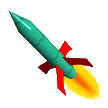Rocket Simulations

## Related Pages

• Rocket Simulations 2D: Launch Angle -- how to run the simulation for launching the rocket at an angle. Warning: you should be familiar with the one-dimensional simulation (this one) before attempting to tackle the 2D version.
• Rocket Equations: direct analytical solution for velocity and altitude - compute peak altitude without the need for simulation.## Numerical Simulation to the Rescue!

Running a simulation of your rocket flight is a great idea, especially if you aren't that familiar with the physics of a rocket flight and you want to learn more about it. Numerical Simulation is easy to understand and easy to set up, especially if you have a spreadsheet like Excel to use. The simulation makes it easy to see what's going on throughout the rocket's flight. Moreover, it may be the only way to really solve the problem you're trying to solve.

I've written this page as if you're running the simulation with a spreadsheet, mainly since that's how I usually do it. However the algorithms are identically the same if you're writing it as a program in C (or Basic, or Java, or whatever).

There are two things about a rocket's flight that make it tough to solve for velocity and altitude:

• first of all the rocket's mass is changing, and
• secondly the drag force changes with the rocket's speed.

If you neglect the changes happening with one factor, you can use calculus to deal with the changes in the other, as I do on my Rocket Equations page. But to figure for both, even calculus isn't much help. That's when Numerical Simulations can come to the rescue.

You should use Numerical Simulation if

• you're planning a very high-powered or high-altitude flight, where rocket mass and/or air density is going to change by more than 50%
• you want to compute the speed of your rocket at the end of the launch rod - during the early part of the burn when thrust is changing radically
• you want to plot a graph of the entire rocket flight, not just solve for a single number.

It's also a good idea to use Numerical Simulation if you don't much feel like messing with calculus.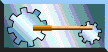## How Numerical Simulation Works

To run a numerical simulation, we break up the time of the rocket's flight into little itty-bitty time periods, calculate velocity and altitude change over each time period, and add them up to get the result. It's really very simple.

So why are we doing it like that?

• Our problem with calculating the rocket's speed or altitude is that numbers we use in the calculation (rocket mass and drag) keep changing on us.
• In numerical simulation, we look only at a very short period of time. During that time, we assume those changing factors are constant.
• We can choose the short period as short as we want, in order to make the assumption true - that those changing factors don't change (much).
• We calculate the new speed and altitude at the end of that short time, then
• use those values, along with new mass and drag values, in the calculations for the next time period.

If I expect the rocket to fly for 10 seconds, and the short time period I choose is 0.1 seconds, then I have to do 100 calculations! That's why numerical simulations and computers go together - the idea is simple but if you do it by hand it's a LOT OF WORK. With the computer it's not a lot of work at all - I wrote and ran my first simulation in 15 minutes.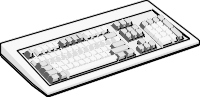## How to Write Your Numerical Simulation

I'll give you a summary of the equations for simulating a rocket's flight first, then I'll explain the calculations one by one. I usually set up a flight simulation in a spreadsheet by creating one column for each of the variables described below. Each row in the spreadsheet then represents a time period, showing the value of all the variables for that time. If you're programming in C (or whatever) each row represents one iteration of the simulation loop.

Note that I call the length of the itty-bitty time period "dt". Then the columns of the spreadsheet (the variables) are as follows:

That's it! Sometimes when I'm getting fancy I may add a column for rho, the air density, so I can change it with altitude, and if it's a multistage rocket with different diameters, I might add a column for A, the area of the rocket. Of course, it's your simulation, you can add any columns you want and vary some other interesting things.

Set your initial conditions by setting the first row of your spreadsheet to all zeroes, except for the "Mass" column, which should be set to your fully-loaded rocket's mass. Then the second row and all rows thereafter have the formulas described above and detailed below.

Now let's look at those variables and equations in more detail.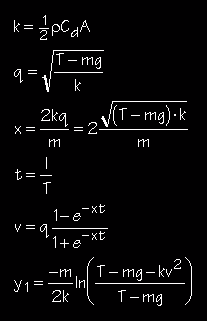## Working with the Equations

### Using Metric

The physics works most easily when working in metric, so convert first before putting numbers into the equations. By metric I mean working strictly in meters, kilograms, and Newtons - no millimeters, centimeters, grams etc.

Some essential conversions to get the job done:

• Multiply inches by 0.0254 to get meters
• Multiply ounces by 0.0284 to get kilograms

I set up constants for the equations at the top of my spreadsheet so I have easy access to them and can change them globally with a single entry change. I also use these cells to compute the conversion to metric

• Rocket Mass, empty (Mr): since I normally have the rocket's empty weight in ounces, I set this cell equal to weight*0.0284 to get the mass in kilograms.
• Engine Case Mass (Me): You can go to the Estes or Aerotech catalogs to get these numbers. They normally give you the fully loaded motor mass and the propellant mass. I set Me to the loaded mass minus the propellant mass. In the past I have used the weight of the burned-out Estes engine cases for Estes motor simulations. I weighed numerous burned-out standard Estes engine cases and got 10.7 grams, or 0.0107 kg.
• Propellant Mass (Mp): this number is readily available from the Estes catalog or the Aerotech catalog, and also from Magnum Rockets' sites on motors. You can also estimate it, as described on my Rocket Equations page, by dividing the impulse of the engine by 800 for black powder motors, or dividing by 1800 for composite motors. This gives you the mass of the propellant in kilograms.
• Impulse (Im): the impulse of your motor in Newton-seconds.
• Thrust (Th): the thrust of your motor in Newtons.
• Burn Time (tb): derived as tb=Im/Th
• Air Density (rho): equal to 1.22 kg/m^3 at sea level, and drops by 10% for each 1000 m of altitude for the first 14 km (46,000 ft). So if you're going much above 1000 m (3300 ft), you may want to create another column for rho (make it a variable for each time period) and use the equation rho=1.22*0.9^(Y/1000) where Y is altitude in meters. Otherwise 1.2 is plenty good enough.
• Drag Coefficient (Cd): this characterizes the relative drag for the shape and smoothness of your rocket, the lower the better. The average rocket has a Cd=0.75, a really sleek one might have a Cd of 0.6 or less, a poor one could easily be 0.9. See John DeMar's excellent Model Rocket Drag Analysis for more details.
• Rocket Diameter (Dr): this is normally given to you in inches, so I set this cell equal to (diameter in inches)*0.0254
• Rocket Area (A): equal to pi (3.14159) times the radius squared, where radius is half the diameter of the rocket (at its widest point). Therefore A = pi*(Dr/2)^2. If you have a multiple stage rocket with more than one diameter, you may want to create a column for area and change it at the appropriate burnout.
• Time Increment (dt): the length of the itty-bitty time periods for which each calculation is done. For example, if I want a calculation done every tenth of a second, this is 0.1; for every hundredth it would be 0.01.
• Mass Decrement (dm): the amount of propellant burned during each time period, therefore dm=Mp/tb (Note how I use it below)
• Gravitational Constant (g): the acceleration that everything feels in the earth's gravitational field, near its surface = 9.8 m/s^2. Following Newtons Second Law, Force=Mass*Acceleration, the gravitational force on your rocket is F = M*g.### Time & Mass

These two columns are easy - simply start with the first row of the time column at zero and the first row of the mass column at Mr+Me+Mp.

Then the formula for each row thereafter in the time column is = (the cell above)+dt.

Likewise in the Mass column is the formula is = (the cell above)-dm, or the equivalent formula, Mr+Me+Mp-T*dm. In the mass column you want to remember to stop subtracting mass at burnout. If you're good with a spreadsheet you can let the spreadsheet do the remembering for you, using an IF statement in the formula. In Excel, the way this works is

IF(statement,formula 1,formula 2)

where if statement is true, then the cell is equal to formula 1, otherwise it's formula 2. So in our case we can get the rocket mass to automatically be correct by using the formula "IF(T<tb,Mr+Me+Mp-T*dm,Mr+Me)". This means that as long as the time is less than the burnout time, subtract (time * mass decrement) from initial rocket mass; after time exceeds burnout time, rocket mass is empty mass plus burned out casing.

What makes using the "IF" statement so great is that once you write the formula you can just copy down like any other column, and forget about where tb occurs. Also if you change thrust and impulse, tb is automatically recalculated (in the constants section above), and mass decrement stops at the new tb automatically in the simulation. In addition - if you calculated your propellant mass using Im/800, (or Im/1800) then when you change impulse, the new propellant mass and mass decrement are automatically calculated for you, and you don't have to worry about changing them, either. Too easy.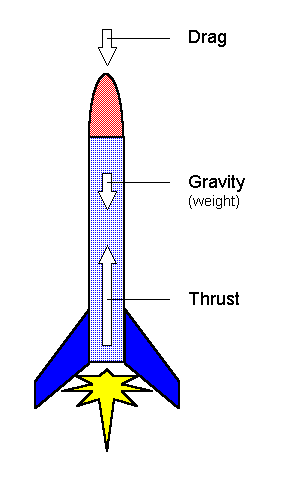## Forces

Rocketry uses the metric measure of force, the Newton. A Newton is about a quarter pound - 0.2248 pound to be precise.

There are three forces acting on your rocket: the motor's thrust pushing it up, gravity pulling down, and drag slowing it down no matter which way it's going.

Thrust of your engine is given by the motor specifications. For example, a C6-5 motor provides 6 Newtons of thrust, or about a pound and a half. You can set this value up in the constants, then using the "IF" statement as we did before, the formula is

IF(T<tb,Th,0)

that is, before burnout time, the thrust is Th (the value we set up in the constants), and after burnout time it's zero.

In reality the thrust is not constant during the burn but it varies. This usually has little effect on the overall altitude reached but has a very significant effect on the early part of the flight. For including variable thrust effects see below.

Gravity: The force of gravity is a constant, downward and therefore negative force. It's negative since it drives the rocket downward and hence is always trying to subtract from its altitude. As Galileo showed by dropping things off the leaning tower of Pisa, the acceleration that gravity produces is the same for all objects no matter what their mass, equal to 9.8 meters/sec/sec (see the Physics page). We call this constant "g" and as I mentioned above the force due to gravity is F=M*g. That says the force due to gravity, that is, the weight of an object (like your rocket) in Newtons is 9.8 times it's mass (in kilograms).Drag Force: drag is simply wind resistance, the force felt when the rocket pushes through the air. The trick to wind resistance is that it always pushes back against the direction of travel, whether the rocket is going up or down (or sideways). That means it's a downward (negative) force during ascent, and an upward (positive) force during descent. Unfortunately drag goes as velocity squared, so the sign of the velocity does not automatically compensate because it's always positive after squaring. You can fix that though, by explicity adding the sign of the velocity to the drag force formula. In Excel it looks like this:

Fd = 0.5*rho*Cd*A*SIGN(V)*V^2

This prevents you from having drag actually accelerate your simulated rocket after it passes apogee and begins to descend. Note that in C and Basic the "sign" function is called "sgn", in Java 1.1 it's called "signum" (using java.math.BigDecimal). Some languages (Java 1.02 and Pascal, for example) don't have it and you have to use V/abs(V).

Remember to use the velocity from the previous time interval (row), otherwise your spreadsheet will correctly complain about circular references, meaning you're trying to compute velocity from drag which you're trying to compute from velocity. Don't work.

Total Force: the acceleration of the rocket is the result of the sum of all the forces acting on the rocket. As shown in the diagram, these forces are thrust in the positive direction, gravity in the minus direction, and drag in the minus direction, so the total force on the rocket is

F = Ft - Fd - M*g

If you have the thrust go to zero after burnout, and if you used the drag formula as I've shown above, the same formula can be set up in the second row (after the initializing first row) and just copied down to the last row.### Sir Isaac Newton at the Wheel

Once you've got the forces and rocket mass figured out, then acceleration, velocity, and altitude fall out quite simply from Newton's Second Law and basic physics. For example, acceleration is determined by solving F=ma for acceleration, yielding a=F/m, which is where our formula above Acc=F/M comes from.

With acceleration determined, the change in velocity during the time period is Acc*dt, so the velocity at the end of the time period is the velocity from the previous time period + Acc*dt. This velocity is in meters/second. You can create a separate column to calculate V*2.237 to see the speed in miles per hour.

With velocity V determined, the change in altitude during the time period is basically V*dt, so the altitude is the altitude from the previous time period + V*dt. This formula works great and if you are not that familiar with Physics it's easy to understand.

If you are familiar with basic physics you might have noticed that the above equation can be refined but my experience is that refinements don't change the result much. In case you're curious, a slightly more accurate (and technically more correct) version is to use previous row's altitude plus previous row's V*dt + 0.5*Acc*dt^2 (where Acc is from this row).

### Assorted Topics

Parachutes: adding a parachute to your simulation is incredibly easy. The drag of the parachute obviously dominates the drag of the rocket, so at the ejection charge time, change the area from that of the rocket to that of the parachute. The drag formula will take it from there for you. If you are using a parasheet, (for example, a flat sheet of plastic) you can keep the Cd of 0.75. If you are using a true, dome-shaped parachute (most people don't) you can change the Cd to 1.5 (per Stine's Handbook of Model Rocketry).

Variable Thrust Curve: When thrust curve data are available, you can set it up in the spreadsheet. Create a little table with times in one column, and thrust values in the next column. If you name the resulting table "Thrust", then the Excel formula for using the thrust curve is

VLOOKUP(T,Thrust,2,TRUE)

where the T is the time value to look up (the first column of the Thrust table), Thrust is the table to look in, 2 means return the matching value in the second column, and "TRUE" is telling the VLOOKUP command that if it doesn't find the exact value for T, take the next lowest entry in the table.

You can do a little trick to enable you to interpolate values and "smooth out" your curve. Instead of a two-column table, make a four column table with two columns for time and two for thrust. The first column is the time values from the thrust curve, the second is the first column copied over and shifted up by one row. Likewise, the third column is the thrust values, and the fourth is the thrust values copied over and shifted up by one row. To keep them straight you may want to write "t" at the top of the first column, "t next" on the second, "thrust(t)" on the third column and "thrust(t next)" above the fourth. Now if you label the whole table (all four columns) "P", you can interpolate by setting the thrust value for time T to be

Ft = vlookup(T,P,3)+ (vlookup(T,P,4)-vlookup(T,P,3)) *(T-vlookup(T,P,1))/ (vlookup(T,P,2)-vlookup(T,P,1))

Well it's ugly but it works. This is one area where a programming language is much prettier, but then when you have to write the user interface and plotting capability programming languages lose luster.

## Looking for Examples?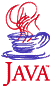My Java Rocket Simulator: a running simulator and the source code used to write it. The simulator will only run if your browser is Java Enabled but you can see the source as an example in any case.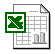My Astrocam Excel SimulationMy MiniMagg Excel Simulation showing how to use thrust curve data to improve simulation accuracy.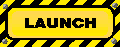Mark Sullivan's Model Rocket Altitude Predictor: an outstanding, working online rocket simulator that does not require Java to run.

## Questions

Your questions and comments regarding this page are welcome. You can e-mail Randy Culp for inquiries, suggestions, new ideas or just to chat.
Updated 19 July 2009